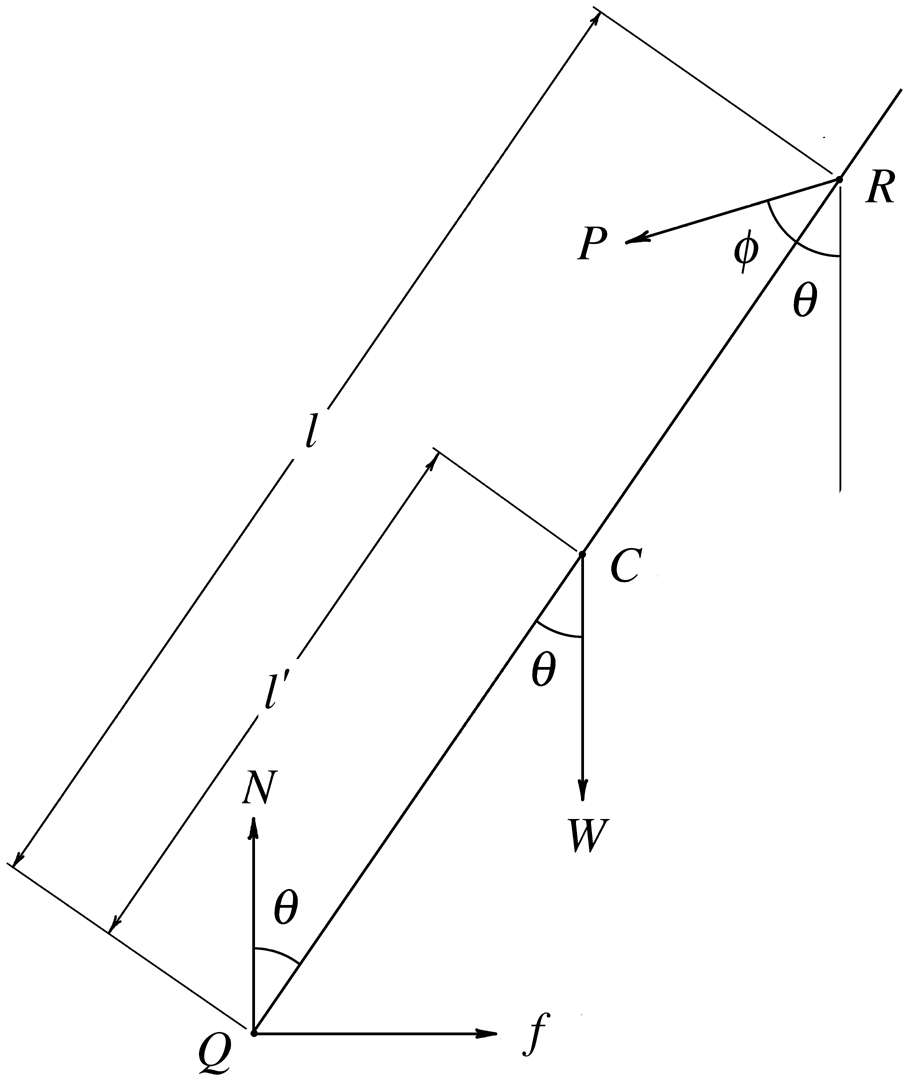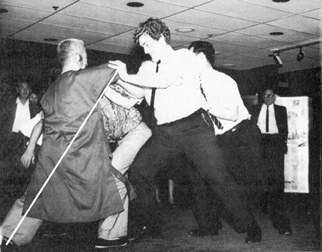T’ai-Chi “Rooting and Redirecting”

How can a small, elderly practitioner withstand a large incoming force without being moved? We will use elementary physics to reveal the mechanical factors involved. Then the internal factors will be discussed.

In addition to showing their skill by doing solo movements, push-hands, and self-defense applications, T’ai-Chi practitioners also demonstrate “rooting and redirecting.” That is, a master will stand in a 70-30 stance and pair off with one or more large, strong partners and ask them to push his body or outstretched arm as forcefully as possible. The goal is for the master to be so rooted that no movement or loss of balance occurs no matter how hard he is pushed (see video of Chen Xiaowang demonstrating rooting).

My first T’ai-Chi teacher, Cheng Man-ch’ing, was known for demonstrating such rooting by standing in the “Ward Off” posture and having one or more partners push his outstretched arm (Fig. 1), thereby showing not only rooting but also peng-jin skills (see article on peng and jin).Fig. 1. In the above photograph, people are pushing Cheng Man-ch’ing’s extended arm. Prof. Cheng was renowned for not collapsing his arm and not moving his feet during such demonstrations. The largest person pushing is the late Patrick Watson.*

Analysis

In the diagram in Fig. 2, line QR, tilted with respect to the vertical at an angle θ, represents the core line of the person being pushed. The effect of the forward leg is neglected because the supportive effect of that leg disappears when the receiving person starts to lose rooting, which is the limiting case being considered. In fact, some practitioners actually lift their forward leg off the ground while demonstrating rooting.Q = the center of the receiving person’s rear foot R = the point of contact of the pushing person f = the force of friction of the floor on the receiving person’s rear foot N = the upward force supplied by the floor C = the center of mass of the receiving person W = the force of gravity on the receiving person (his weight) P = the magnitude of the force of the person pushing φ = the angle between the line QR and the direction of P θ = the angle between the line QR and the vertical l = the distance from Q to R l′ = the distance from Q to C

Fig. 2. Forces on a person who is demonstrating rooting. QR represents the line from the center of his rear foot to the point of applied force.

Analysis of Forces

We will assume that the person being pushed is in both rotational and translational equilibrium. Then we will investigate the effects produced when different parameters are varied and draw conclusions.

For a body to be in translational equilibrium, the sum of all forces must equal zero. For a body to be in rotational equilibrium, the sum of the torques of all forces about any point must equal zero. Torque (the rotational effect of a force), is represented by the Greek letter τ. The torque τp of a force F about a point p is calculated as follows: Any line is drawn from p to the line of F. The torqe about p then equals the distance from p to F along the drawn line, multiplied by the component of F perpendicular to that line. Note that the result does not depend upon which line is drawn. Moreover, the component of F parallel to that line has no rotational effect.

The torque of a force is usually considered to be positive if its rotational effect is counterclockwise and negative if its rotational effect is clockwise.

Rotational Stability

Setting the sum Σ of torques about Q = 0 (see Fig. 2):

ΣτQ = 0

l P sin φl′ W sin θ = 0

Therefore,  P = l′ W sin θ ÷ l sin φ.   (Eq. 1)

Note that l, l′, and W are constants. Thus, assuming continuing stability of the person being pushed, we will investigate the effect of different values of θ and φ on the magnitude P of the force exerted by the person pushing. The larger P, the greater the force exerted by the person pushing without loss of stability of the person being pushed.

Conclusions

Looking at Eq. 1, we see that as θ increases, so does P because, as θ increases from 0° to 90°, sin θ increases from 0 to 1. Thus, extending your rear leg as far back as possible and leaning forward increases θ, thereby increasing your stability due to the effect of your weight.

Also, as φ increases from 0 to 90°, sin φ increases from 0 to 1. Because sin φ is in the denominator, the smaller φ, the larger P without the person being pushed losing stability. In fact, as φ approaches zero, P approaches infinity! The extreme condition (P = infinity) means that no matter how much force a person exerts on you along the line QR, your stability will be maintained.

Friction with the Floor

The effects of W, θ, and φ on the frictional force f of the floor are found by setting the horizontal components of all forces to zero:

ΣFx = 0

Assuming no slipping,

fP sin (θ + φ) = 0

Therefore,  f = P sin (θ + φ). (Eq. 2)

Setting the vertical components of all forces to zero:

ΣFy = 0

NWP cos (θ + φ) = 0

Therefore,  N = W + P cos (θ + φ).   (Eq. 3)

Also, the maximum frictional force is given by fmax = μsN, where μs is the coefficient of static (not sliding) friction between the floor and the foot of the person being pushed. μ depends only on the nature of the surfaces (for example, wood and rubber).

Therefore,  fmax = μs [W + P cos (θ + φ)].   (Eq. 4)

Conclusions

From Eq.3, the smaller θ and or φ is, the larger fmax is. That relationship means that the more you extend your rear leg and the more along line QR you are pushed, the greater the frictional force of the floor on your rear foot and the less chance you will slide backward.

The question is how you can regulate the direction of the pusher’s force to advantage. To this end, consider next the reaction to the forces exerted by the person pushing. According to Newton’s third law, if object A exerts a force on object B (the action), then B exerts an equal and opposite force on A (the reaction). Thus, when the person A (the person pushing) exerts a force of magnitude P in a certain direction on person B (the person receiving), then B automatically exerts a force of magnitude P on A in the opposite direction. Thus, if the force of the person pushing has a downward component, the reaction force exerted back on him will automatically have a corresponding upward component. The smaller the angle φ, the more B will be rooted (not losing balance or sliding) and the more A will be losing balance and sliding.

So how can B get A to push along the line RQ (φ = 0)? The answer is for B to exert an upward force on A, thereby causing A to exert a downward reaction force on B. Note in Fig. 3 how Prof. Cheng’s elbow is lifted, causing Patrick Watson to push partly downward. The combination of downward and forward forces exerted by Watson is then a single resultant force directed toward Prof. Cheng’s rear foot, thus causing Prof. Cheng to be more rooted and less likely to slide backward but causing Watson to be less rooted and more likely to slide backward.Fig. 3. Note that the line drawn from the center of Prof. Cheng’s rear foot to the point of contact of Patrick Watson is along his rear leg.

Internal Aspects

In order to be able to withstand another’s force without losing your root requires an unobstructed, direct internal path for force exerted on you to pass to the floor. That state, called sung, is achieved by recreating the super relaxed state you experience after taking a hot bath. The contact point of your partner on your body must be totally relaxed and liquid. Moreover, any active force you exert on your partner must not be through muscular contraction but be, instead, a kind of hydraulic expansion. The feeling is that the water in each of your muscle cells is expanding.

*From T’ai-Chi Ch’uan, Body and Mind, published by the Tai Chi Chuan Association, 211 Canal Street, New York, NY, 1968.

More Articles on and Information About T’ai Chi